# Branch point

singular point of multi-valued character

An isolated singular point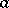of an analytic function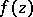of one complex variablesuch that the analytic continuation of an arbitrary function element ofalong a closed path which encirclesyields new elements of. More exactly,is said to be a branch point if there exist: 1) an annulus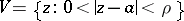in whichcan be analytically extended along any path; 2) a pointand some function element ofrepresented by a power series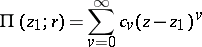with centre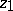and radius of convergence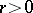, the analytic continuation of which along the circle, going around the path once in, say, the positive direction, yields a new element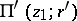different from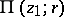. If, after a minimum numberof such rounds the initial elementis again obtained, this is also true of all elements of the branch (cf. Branch of an analytic function) ofdefined inby the element. In such a caseis a branch point of finite order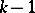of this branch. In a punctured neighbourhoodof a branch pointof finite order this branch is represented by a generalized Laurent series, or Puiseux series: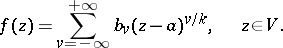(1)

Ifis an improper branch point of a finite order, then the branch ofis representable in some neighbourhoodby an analogue of the series (1):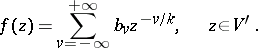(2)

The behaviour of the Riemann surface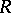ofover a branch point of finite orderis characterized by the fact thatsheets of the branch ofdefined by the elementcome together over. At the same time the behaviour of other branches ofovermay be altogether different.

If the series (1) or (2) contains only a finite number of non-zero coefficients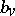with negative indices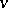,is an algebraic branch point or an algebraic singular point. Such a branch point of finite order is also characterized by the fact that asin whatever manner, the values of all elements of the branch defined byinor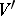tend to a definite finite or infinite limit.

Example: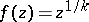, where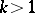is a natural number,.

If the series (1) or (2) contain an infinite number of non-zero coefficientswith negative indices, the branch points of finite orderbelong the class of transcendental branch points.

Example: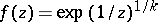, whereis a natural number,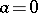.

Finally, if it is impossible to return to the initial element after a finite number of turns,is said to be a logarithmic branch point or a branch point of infinite order, and is also a transcendental branch point.

Example: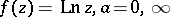.

Infinitely many sheets of the branch ofdefined by the elementcome together over a logarithmic branch point.

In the case of an analytic function of several complex variables,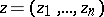,, a pointof the spaceoris said to be a branch point of order,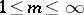, if it is a branch point of orderof the, generally many-sheeted, domain of holomorphy of. Unlike in the case, branch points, just like other singular points of analytic functions (cf. Singular point), cannot be isolated if.